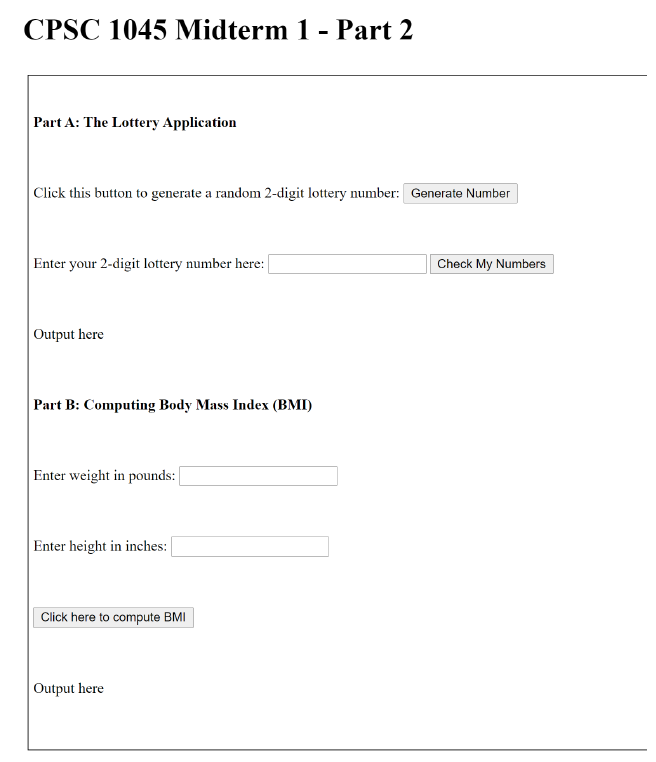Still Thinking Of Assignment Help & Grades ? Book Your Assignment At The Lowest Price Now & Secure Higher Grades! CALL US +91-9872003804

Order Now

Value Assignment Help

# HTML Assignment Help Online

## Please follow the instructions carefully and complete all of the steps.

1.  An HTML file with the name midterm1.html already exists in the Midterm1_Part2 folder you downloaded. It contains all the tags you would need to complete this exam. The document looks as follows

### CPSC 1045 College Midterm I Programming1. In the Midterm1_Part2 folder, create a new JavaScript file named midterm1.js
2.  Inside midterm1.js, write the JavaScript to complete the following tasks.

A. [10 marks]

• a) Create a global variable called lotto that is initialized to 0.
• b) When the Generate Number button is pressed, a function generate lotto() should generate a random 2-digit number and store it in the global variable lotto.
• c) When the Check My Numbers button is pressed, a function compare numbers() should run to see how closely the number entered by the user matches the randomly generated lottery number. The user is a winner in the following three scenarios:
• If the user input matches the lotto number in the exact order, the award is \$10,000.
• If both digits in the user input match both digits in the lottery number, but out of order, the award is \$3,000.
• If one digit in the user input matches a digit in the lottery number, the award is \$1,000.
• d) If the number doesn’t match the lottery number at all, there is no award for the user.
• e) Test that your JavaScript executes correctly. Here are some sample runs:

B. [10 marks]

Write a JavaScript function computeAndInterpretBMI() that takes as input the height and weight of a person and displays a friendly message stating his/her BMI and as to whether the person is “Under-weight”, “Normal”, “Overweight”, or “Obese”.

• a) The user enters his/her weight and height in the provided boxes. The weight should be entered in lbs and converted into kg by multiplying with 0.45359237. Similarly, the height is entered in inches and converted into meters by multiplying with 0.0254.
• b) The formula for calculating the BMI is:
• 𝑤𝑤𝑤𝑤𝑤𝑤𝑤𝑤ℎ𝑡𝑡𝑡𝑡𝑡𝑡𝑡𝑡𝑡 𝑡𝑡𝑡𝑡𝑡 𝑡𝑡𝑡𝑡𝑡𝑡𝑡𝑡 / ℎ𝑒 𝑒 ℎ𝑡𝑡𝑡𝑡𝑡𝑡𝑡𝑡𝑡 𝑡𝑡𝑡 𝑡𝑡𝑡𝑡2
• c) The rules for categorizing a person as “Underweight”, “Normal”, “Overweight”, or “Obese” are as follows:
•  if the BMI is less than 18.5, the person is “Underweight”
•  if the BMI is greater than or equal to 18.5 but less than 25, the person is “Normal”
•  if the BMI is greater than or equal to 25 but less than 30, the person is “Overweight”
•  if the BMI is greater than or equal to 30, the person is “Obese”
• d) The BMI should be displayed with 2 digits of precision after the decimal point. For
• For example, if the calculated BMI is 34.31424464, it should display as 34.31.

Some sample runs of the application are shown below:

1. 1
2. 2

### Latest Blogs from value Assignment helpBlog